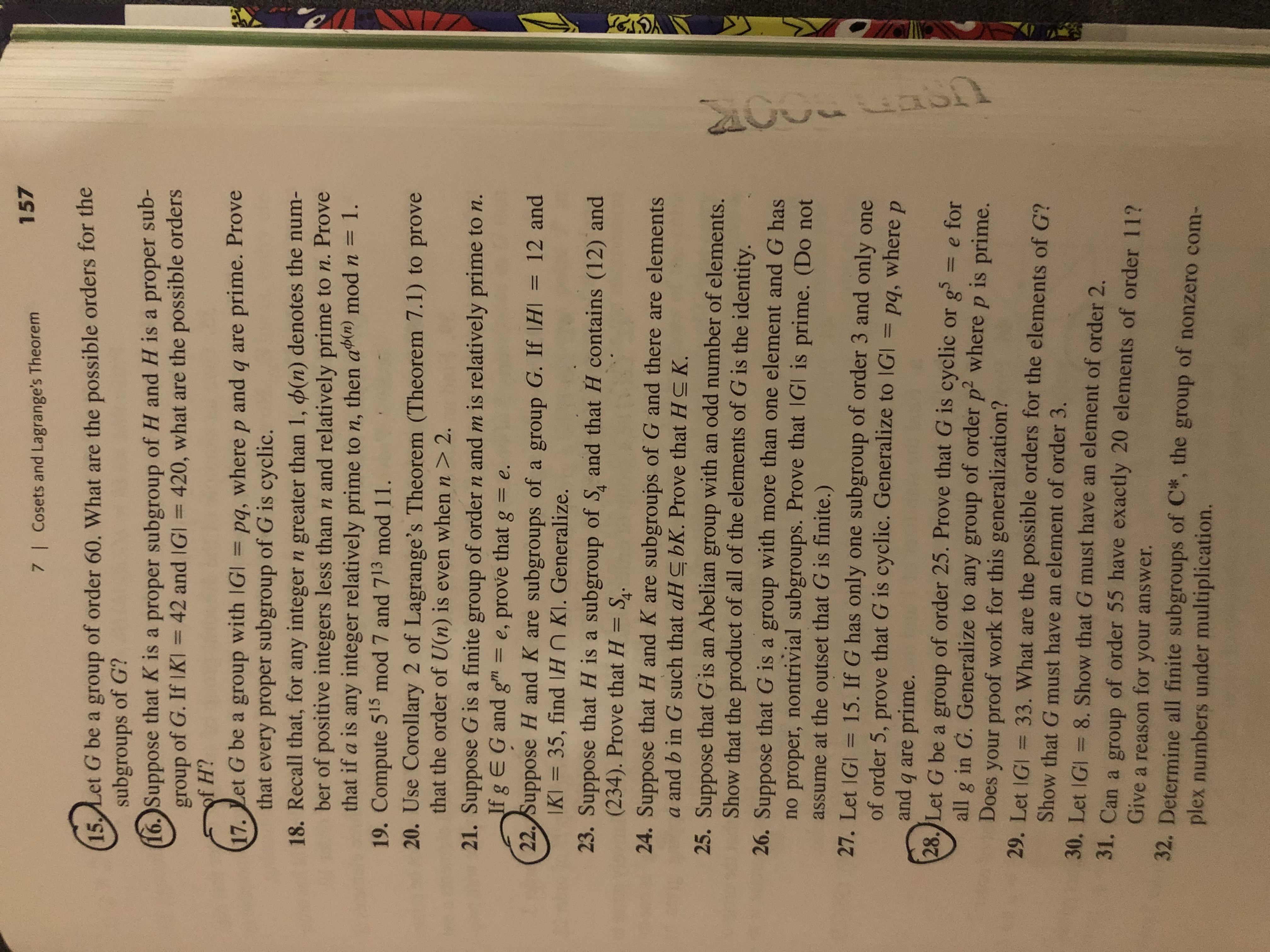1577 I Cosets and Lagrange's Theorem(15 Let G be a group of order 60. What are the possible orders for thesubgroups of G?16.)Suppose that K is a proper subgroup of H and H is a proper sub-group of G. If IKI 42 and IGI 420, what are the possible ordersof H?17. Let G be a group with IGI pq, where p and q are prime. Provethat every proper subgroup of G is cyclic.18. Recall that, for any integer n greater than 1, (n) denotes the num-ber of positive integers less than n and relatively prime to n. Provethat if a is any integer relatively prime to n, then abin) mod n = 1.19. Compute 515 mod 7 and 713 mod 11.20. Use Corollary 2 of Lagrange's Theorem (Theorem 7.1) to provethat the order of U(n) is even when n > 2.21. Suppose G is a finite group of order n and m is relatively prime to n.If g E G and g"m22. Suppose H and K are subgroups of a group G. If IHI = 12 andKI= 35, find H KI. Generalize.23. Suppose that H is a subgroup of S and that H contains (12) and(234). Prove that H S.=e, prove that ge.24. Suppose that H and K are subgroups of G and there are elementsa and b in G such that aHC bK. Prove that HC K.25. Suppose that Gis an Abelian group with an odd number of elements.Show that the product of all of the elements of G is the identity.26. Suppose that G is a group with more than one element and G hasno proper, nontrivial subgroups. Prove that IGI is prime. (Do notassume at the outset that G is finite.)27. Let IGI = 15. If G has onlyof order 5, prove that G is cyclic. Generalize to IGI = pq, whereand q are prime.28. Let G be a group of order 25. Prove that G is cyclicall g in G. Generalize to any group of order p2 where p is prime.Does your proof work for this generalization?29. Let 1Gl = 33. What are the possible orders for the elements of G?one subgroup of order 3 and only onepg5 = e forShow that G must have an element of order 3.30. Let IGl = 8. Show that G must have an element of order 2.31. Can a group of order 55 have exactly 20 elements of order 11?Give a reason for your answer.32. Determine all finite subgroups of C*, the group of nonzero com-plex numbers under multiplication.

Question

16help_outlineImage Transcriptionclose157 7 I Cosets and Lagrange's Theorem (15 Let G be a group of order 60. What are the possible orders for the subgroups of G? 16.)Suppose that K is a proper subgroup of H and H is a proper sub- group of G. If IKI 42 and IGI 420, what are the possible orders of H? 17. Let G be a group with IGI pq, where p and q are prime. Prove that every proper subgroup of G is cyclic. 18. Recall that, for any integer n greater than 1, (n) denotes the num- ber of positive integers less than n and relatively prime to n. Prove that if a is any integer relatively prime to n, then abin) mod n = 1. 19. Compute 515 mod 7 and 713 mod 11. 20. Use Corollary 2 of Lagrange's Theorem (Theorem 7.1) to prove that the order of U(n) is even when n > 2. 21. Suppose G is a finite group of order n and m is relatively prime to n. If g E G and g"m 22. Suppose H and K are subgroups of a group G. If IHI = 12 and KI= 35, find H KI. Generalize. 23. Suppose that H is a subgroup of S and that H contains (12) and (234). Prove that H S. = e, prove that g e. 24. Suppose that H and K are subgroups of G and there are elements a and b in G such that aHC bK. Prove that HC K. 25. Suppose that Gis an Abelian group with an odd number of elements. Show that the product of all of the elements of G is the identity. 26. Suppose that G is a group with more than one element and G has no proper, nontrivial subgroups. Prove that IGI is prime. (Do not assume at the outset that G is finite.) 27. Let IGI = 15. If G has only of order 5, prove that G is cyclic. Generalize to IGI = pq, where and q are prime. 28. Let G be a group of order 25. Prove that G is cyclic all g in G. Generalize to any group of order p2 where p is prime. Does your proof work for this generalization? 29. Let 1Gl = 33. What are the possible orders for the elements of G? one subgroup of order 3 and only one p g5 = e for Show that G must have an element of order 3. 30. Let IGl = 8. Show that G must have an element of order 2. 31. Can a group of order 55 have exactly 20 elements of order 11? Give a reason for your answer. 32. Determine all finite subgroups of C*, the group of nonzero com- plex numbers under multiplication. fullscreen
Step 1

By Lagrange's Theorem, |K| divides |H|, since K is a proper subgroup of H. Given that |...

Want to see the full answer?

See Solution

Want to see this answer and more?

Our solutions are written by experts, many with advanced degrees, and available 24/7

See Solution
Tagged in

Math